# Special Feature

Teaching Mathematical Modelling
in Singapore Schools

Ang Keng Cheng
National Institute of Education

Introduction

The purpose of this paper is to examine the possibility of introducing the teaching of mathematical modelling to the secondary school curriculum in Singapore.  As well, the benefits of teaching and learning mathematical modelling are discussed.  Examples to illustrate the process of mathematical modelling using only basic mathematical ideas and concepts are presented.  These serve to illustrate that school mathematics can be used to provide experiences of the process of mathematical modelling in the classroom.  Some implications on the teaching and learning of mathematics using such an approach are examined and discussed.

One of the primary aims of mathematics education for secondary schools in Singapore is to enable pupils to acquire the necessary mathematical knowledge and skills and to develop thinking processes so as to apply them in mathematical situations in real life.  In fact, the mathematics curriculum in Singapore is designed around a framework with “Mathematical Problem Solving” as the central focus (Ministry of Education, 2001).

In principle, with mathematical problem solving as a theme, the curriculum is expected to focus on applying mathematics in practical situations and real life problems.   In the process of delivering the curriculum, it is hoped that mathematics learners will not only appreciate the beauty of the subject but also the usefulness and power of mathematics.

In practice, however, the emphasis has been on solving routine mathematical problems in a context-free environment.  Even on the odd occasion when a “real life” problem or example is discussed in the classroom, it is typically a rather artificial problem created for the purpose of fitting it into the topic in question.  The problem is usually complete by itself, and is presented in a very clean and tidy state.  Such practice makes it difficult to convince the learner that real life applications of mathematics do indeed exist.

In addition, mathematics has often been thought of by pupils as consisting of a set of distinct topics that are compartmentalised and self-sufficient.  In real life, however, problems tend to transcend a number of disciplines and are often not so well defined.  Often, we need to apply ideas and concepts in one area to solve problems arising in another.  Mathematical modelling offers excellent opportunities to connect and use ideas from different areas.

The rapid development of computers and their availability in the classroom have had a tremendous impact on the expectations of the computational skills of all students.   Skills thought to be essential in the mathematics curriculum may not be so in future (Arora and Rogerson, 1991).  However, applications of mathematics in problem solving and mathematical modelling should remain an integral part of the curriculum, because these will always be useful.

## What is Mathematical Modelling?

Mathematical modelling is a process of representing real world problems in mathematical terms in an attempt to find solutions to the problems.  A mathematical model can be considered as a simplification or abstraction of a (complex) real world problem or situation into a mathematical form, thereby converting the real world problem into a mathematical problem.  The mathematical problem can then be solved using whatever known techniques to obtain a mathematical solution.  This solution is then interpreted and translated into real terms.  Figure 1 shows a simplified view of the process of mathematical modelling.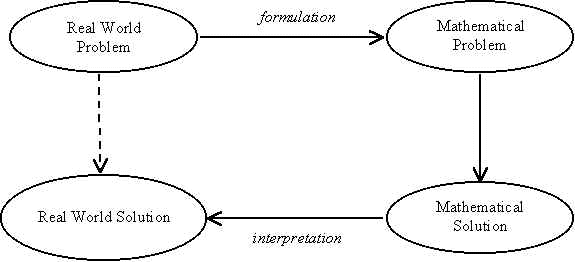Figure 1: A simple view of the mathematical modelling process

The above is, of course, a grossly simplified definition for the usually complex process of modelling.  However, for the purpose of the present discussion, it is sufficient to note that in mathematical modelling, the starting point is a real world problem or situation.

As we shall see, in mathematical modelling, the emphasis is in solving a problem rather than finding an answer that must exist.  Sometimes, we may not even be able to solve the problem entirely, although we hope to move one step closer to obtaining a solution.  At other times, we are happy with a good approximation to the solution of the problem when an “exact answer” either does not exist or is beyond reach.

Hence, when we approach the teaching of mathematics through mathematical modelling, we are really teaching mathematical problem solving.  We present mathematics in action, instead of as a confusing set of formulae scribbled on the chalkboard.  We place mathematics in some context and focus on why mathematics exists in the first place.  Moreover, many challenging and exciting skills are used in developing models and these have often been ignored in traditional school mathematics (Abrams, 2001).  Some of these will become apparent in the next section when we examine specific examples.

Examples

In this section, examples of how the process of mathematical modeling may be introduced in the classroom using basic mathematical ideas and concepts are presented.  Some of these examples are adapted from sources such as Swetz and Hartzler (1991), Wood (1992), and Blane and Evans (1993).

Example 1: The linear function (or graph of y = mx + c)

A common way of introducing the linear function is to use the graph of a straight line.  One would normally state that the graph of the linear function y = mx + c is a straight line with gradient m and y-intercept c.   This context-free way of teaching is efficient and neat.  However, it may be more interesting to see how such a graph and function can actually arise from a real practical situation.

Consider the following situation where water flows from a tap into a measuring cylinder at a constant rate (as depicted in Figure 2).  Suppose we wish to construct a model to show how the water level changes with time so that we can predict how long it would take to fill the whole cylinder.  The water level at various points in time can be read off the measuring cylinder.  The data is recorded in the form of a graph as shown.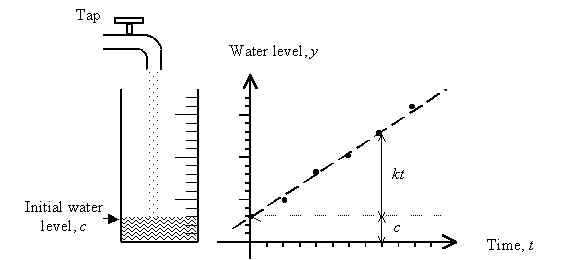Figure 2: Representing rise in water level using a linear function

From the data, we can now try and guess the relationship between the water level,y, and the time after the tap is turned on, t, assuming that the initial water level is c.  It is not hard to see that the water level, y, at any time t should be c plus some positive number, and this positive number should depend on t.  Eventually, the model obtained should look something like

y = c + kt.

By modelling this simple physical situation, the linear relationship could “come alive”.  The linear function is given some context and the graph actually represents something real and physical.   Furthermore, the process of modelling would hopefully enable the learner to appreciate other related concepts.  For instance, we get a steeper gradient of the graph when the rate of water flowing from the tap is increased.

Example 2: The car park problem

A typical school compound would normally have some car park space.  The parking lots are usually already painted, lines drawn and so on.  Suppose we wish to check to see if the existing plan has made maximum use of the car park space.  If not, we wish to re-design the space to increase the number of lots.

Such a problem would involve questions like the following:

• How many cars can be parked along one curb using parallel parking?
• How much space should there be for traffic within the car park?

For angle parking, we could consider the relationship between the curb space, x and the angle the lines make with the curb, q (See Figure 3).  We can first assume that a typical width, w, for a lot is 2.5m.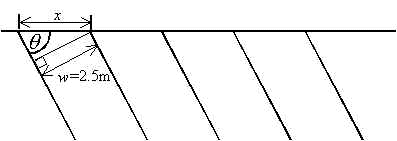Figure 3: Car park problem

It is not hard to see that the relationship between the three variables, x, q and w is.

Suppose we fix the lot width (w) and wish to see how the curb space varies with the angle of the lot.  Using a simple spreadsheet, we can generate a table of values (see Table 1) and its graph (see Figure 4) as follows:

 Table 1: Values of q and x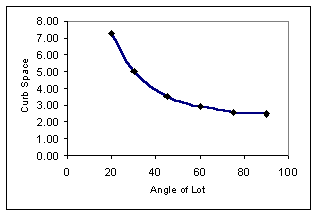Figure 4: Graph of curb space against angle of lot q x (in m) 20 7.31 30 5.00 45 3.54 60 2.89 75 2.59 90 2.50

One can then use the information to make a decision on the car park design, and hopefully answer the questions posed.

Example 3: Biggest box problem

Suppose we intend to make an open-top box using a square piece of card of side s by cutting a square (of side, say x) from each corner of the card (see Figure 5).  The resulting piece is then folded to form the box.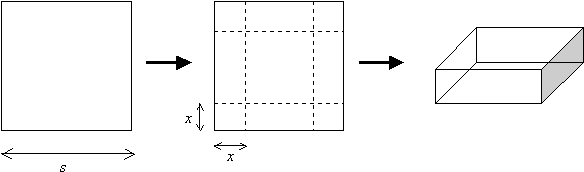Figure 5: Biggest box problem

The question is: what should x be if we wish to make the biggest box (in terms of volume)?

There are several approaches to this problem.  Here, two are described.

a)      Empirical approach

The empirical model involves actually constructing the boxes and taking measurements.  This has to be done systematically just like in performing a scientific experiment.  Since we are particularly interested in the relationship between the size of the smaller square (i.e. x) and the volume of the box, we systematically make boxes using different values of x.

The sides of the box can then be measured and volume calculated for each case.  Alternatively, the volume may be estimated by first pouring sand to completely fill the box.  The amount of sand used can be measured using a measuring cylinder.  Still another variant could be to weigh the sand instead.  Whichever approach is used, the results can be presented in the form of a graph (Figure 6):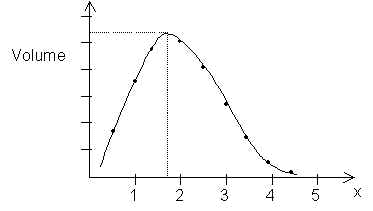Figure 6: Graph of Volume against x (from data)

A “curve of best fit” is then sketched to locate and estimate x that gives the maximum volume.

b)      Analytical approach

An analytical or theoretical model may also be constructed to solve the problem.  This approach is more abstract and involves the use of algebra and geometry.  We model the box by a geometric diagram (such as the one in Figure 5).   We then find the volume of the box in terms of the dimensions s and x.  It is not hard to see that the volume of the box, V is given by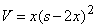, or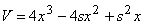.

Suppose the original square cards have sides of dimension, say, s = 10 cm.  Then, we have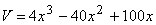.

This is perhaps a good point to introduce the cubic function.   In this particular case, the function models the relationship between the volume of the box and the size of the cut-off square.   It now remains for us to find the value of x that makes V maximum.   How this is done depends on the mathematical ability and maturity of the learner.  For instance, a student familiar with calculus may choose to find the derivative and the turning point of the function to obtain the maximum.  Another may use a graphing tool to plot a graph of V against x to estimate the maximum.  Figure 7 shows a plot generated from the popular graphing tool, Graphmatica.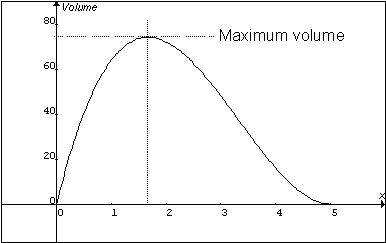Figure 7: Graph of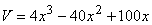(from graphing tool)

Example 4: Modelling population growth

A classic example of modelling with first order ordinary differential equations is the population growth model.  The Malthus model (or exponential growth model) is often used as an introductory example to modelling of populations.  In this case, if P(t) is the population of a species at any time t, then the differential equation is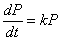for some constant, k.

However, this is not a satisfactory model as it implies that the population will grow exponentially without bounds.  A more realistic model is the Verhulst model, which incorporates competition into the equation.  The Verhulst model, also known as the logistic equation, is given bywhere r and K are positive constants.  In this case, r is the intrinsic reproduction rate and K is the carrying capacity of the population.

It may seem difficult at first to discuss such population models before a basic course in calculus.  However, it is possible to introduce a discrete analogue of the model.  This takes the following form: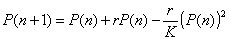where n is an integer.

It is somewhat difficult to obtain actual data to motivate such population models.   One could perform experiments (e.g. using yeast culture) and collect data to represent the population at each stage.  Alternatively, data can be obtained from other sources, such as the Internet, or from the literature.  For instance, Figure 8 shows a set of data from a study on a Barnacle Goose population (Armson, Cockroft and Stone, 2000).  The curve is obtained using the logistic equation and provides a model for the variation of goose population with time.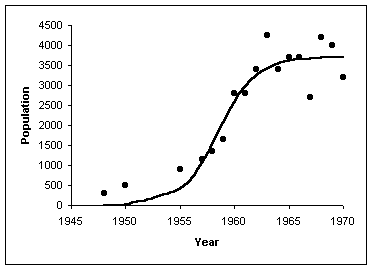Figure 8: Barnacle Goose population (adapted from Armson et al, 2000)
(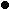: actual data,: model)

Example 5: Numerical estimation

In the previous example, we discussed the model for, say, a goose population.   In the process, we need to estimate the number of geese at some time.  It would be interesting to see how this is done as it is virtually impossible to actually count the number of geese in a region given that such a region is usually very big.

In this example, we examine how an ecologist estimates the population of a particular species of animals.  The mathematics applied here is simply the idea of ratio and proportion.

Suppose we are able to trap and tag x1 number of geese initially.  These are then released to join the rest of the population.  After a lapse of time (to allow “mixing” of tagged and untagged geese), another, say, y number of geese is trapped.  Of these, we count the number that have been tagged previously, and let this number be x2.

Suppose conditions are more or less the same at both occasions when the geese were caught.  We can then assume that the proportion of tagged geese at both occasions should be roughly equal.  That is,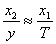where T is the total number of geese in the region.  We thus have an estimate for the geese population.

This simple example not only illustrates the usefulness of the concept of ratio and proportion but also gives it a practical context.

## Implications

Teachers who are keen on introducing mathematical modelling in their mathematics lessons would need to look for ideas.  There are resources, though these are not as readily available as standard textbooks.  Often, it is this lack of ready resources and material that creates a resistance towards teaching mathematical modelling.  Educators will need to put together a more problem-centred set of instructional material.  Teachers may need to be more resourceful when preparing lessons, and look beyond the standard text for suitable and appropriate material.  The challenge is to look for problems that can be adapted according to the level of mathematical ability and maturity of the learners.  Examples of such resources are Burghes, Galbraith, Price and Sherlock (1996) and Saaty and Alexander (1981).

Apart from resources, some modelling skills and understanding of the processes involved are essential in teaching it successfully.  A professional applied mathematician would normally have some experience of one kind or another.   Providing parallel experience for teachers is not easy.  However, it is possible for teachers to learn alongside their pupils.  Alternatively, teachers could attend in-service training to gain such experience (Eyre and Thompson, 1987).

One also needs to realise that there is a distinct difference between teaching mathematical models and teaching mathematical modelling.  Whereas in the former the emphasis is on the product (the models), in mathematical modelling, the focus is on the process of arriving at a suitable representation of the physical, real world situation.  One begins with a real problem and progresses step by step towards possible solutions.

Mathematical modelling also provides an excellent platform for studies and experiments of an inter-disciplinary nature.  Problems may arise (and they usually do) from other disciplines.  This provides the mathematics teacher with excellent opportunities to collaborate with other teachers.  With the Ministry of Education’s current emphasis on inter-disciplinary project work, mathematical modelling may be an essential component of the curriculum.

Another concern that teachers may have is whether mathematical modelling is for students of all abilities.   While it may be true that a student with a stronger mathematics background would be in a better position to appreciate the problems posed, it is possible to provide rich learning environments in realistic context for the average student (Swan, 1991).  Problems can be broken into simpler tasks that encourage exploration and discussion.

## Conclusion

In this paper, the relevance of teaching mathematical modelling as part of the school mathematics curriculum was discussed.  The examples presented are meant to provide illustrations of the process of mathematical modelling using mathematical ideas and concepts that are already in the curriculum.   Thus, there is no need to revamp the curriculum – there is need, however, to rethink our approach to the teaching of some topics in mathematics.

What is taking place in our current mathematics classroom is still a lot of drill and practice on the procedures of solving specific mathematical problems.  While these may be important skills to impart on the mathematics student, the need for routine manual calculations may diminish with time.  Furthermore, with the advent of high speed, high performance computers, the emphasis may no longer be such drudgery.  After all, mathematics is more than just about arithmetic – it is about problem solving.  Teaching mathematical modelling involves high-order thinking skills in representation of the real world, as well as skills of problem solving.  These are desirable outcomes that as important as getting the “right answers” to “problem sums”.

## References

Abrams, J.P. (2001). Teaching Mathematical Modeling and the Skills of Representation, In The Roles of Representation in School Mathematics, 2001 Yearbook, NTCM, (Eds. Cuoco, A.A. and Curcio, F.R.), pp. 269-282.

Armson, R., Cockroft, J.M. and Stone, J.A.R. (2000). Modelling a Barnacle Goose Population, Teaching Mathematics and its applications, Vol.19, No.2, pp.74-82.

Arora, M.S. and Rogerson, A. (1991). Future Trends in Mathematical Modelling and Applications, In Teaching of Mathematical Modelling and Applications, (Eds. Niss, M., Blum, W., Huntley, I.), Ellis Horwood, New York, pp.111-116.

Blane, D. and Evans, M. (Eds).  (1993).   Mathematical Modelling for the Senior Years, Acacia Press, Australia.

Burghes, D., Galbraith, P., Price, N. and Sherlock, A. (1996).  Mathematical Modelling, Prentice Hall International, UK.

Eyre, R.J. and Thompson, D. (1987).  Mathematical Modelling in a College of Education, In Mathematical Modelling Courses, (Eds. Berry, J.S., Burghes, D.N., Huntley, I.D., James, D.J.G. and Moscardini, A.O.), Ellis Horwood, New York, pp.179-187.

Ministry of Education. (2001).  Mathematics Syllabus (Secondary), Singapore.

Saaty, T.L. and Alexander, J.M. (1981). Thinking with Models, Pergamon Press, Oxford.

Swan, M. (1991). Mathematical Modelling for all abilities, In Teaching of Mathematical Modelling and Applications, (Eds. Niss, M., Blum, W., Huntley, I.), Ellis Horwood, New York, pp.137-146.

Swetz, F. and Hartzler, J.S. (Eds). (1991). Mathematical Modelling in the Secondary School Curriculum, NTCM.

Wood, G. (Ed). (1992). Mathematical Modelling in the Senior Secondary School – A Teacher Resource, The Mathematical Association of South Australia Inc., Australia.

_______________________________________

 Graphmatica is a graphing tool developed by ksoft Inc., and available at http://www8.pair.com/ksoft

Note: This article was first published in 'The Mathematics Educator, 2001, Vol. 6, No. 1'.

Ang Keng Cheng is an assistant professor at the National Institute of Education (NIE).  Before joining the NIE, he was a teacher at a junior college in Singapore.  He obtained his PhD from the Adelaide University (Australia) in the area of mathematical modelling.